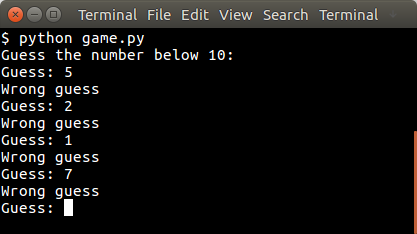# Tag: game

## Jump and run in Pygame

In this article you will learn how to implement jump and run logic in Pygame. To do so, we will implement the player logic.

You may like
Python Game Development for Beginners

Movement
Moving left and right is similar to the previous tutorial and simply mean changing the (x,y) position of the player. For jumping, we use a formula from classical mechanics:

Where F is the force up/down, m is the mass of your object and v is the velocity. The velocity goes down over time because when the player jumps the velocity will not increase more in this simulation. If the player reaches the ground, the jump ends. In Python, we set a variable isjump to indicate if the player is jumping or not. If the player is, its position will be updated according to the above formula.

Full Code:

If you want to jump on objects, simply add them to the screen, do collision detection and reset the jump variables if collision is true.

You may like
Python Game Development for Beginners

## Simple text game with Python

In this article we will demonstrate how to create a simple guessing game.
The goal of the game is to guess the right number.

Example
An example run below:Simple text game with Python

You may like
Python Game Development for Beginners

## Random number

The user will be asked to guess the random number. We first pick the random number:

The randint() function will pick a pseudo random number between 1 and 10. Then we have to continue until the correct number is found:

## Python Guessing Game

The code below starts the game:

An example run:

You may like
Python Game Development for Beginners